Courses

# Test: The Triangle And Its Properties - 2

## 20 Questions MCQ Test Mathematics (Maths) Class 7 | Test: The Triangle And Its Properties - 2

Description
This mock test of Test: The Triangle And Its Properties - 2 for Class 7 helps you for every Class 7 entrance exam. This contains 20 Multiple Choice Questions for Class 7 Test: The Triangle And Its Properties - 2 (mcq) to study with solutions a complete question bank. The solved questions answers in this Test: The Triangle And Its Properties - 2 quiz give you a good mix of easy questions and tough questions. Class 7 students definitely take this Test: The Triangle And Its Properties - 2 exercise for a better result in the exam. You can find other Test: The Triangle And Its Properties - 2 extra questions, long questions & short questions for Class 7 on EduRev as well by searching above.
QUESTION: 1

Solution:
QUESTION: 2

Solution:
QUESTION: 3

### In the Pythagoras property, the triangle must be ___________ .

Solution:
QUESTION: 4

Find the value of x in this figure.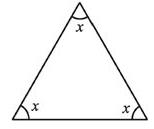Solution:
QUESTION: 5

Find the value of x in given figure.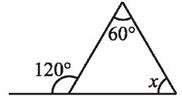Solution:

Exterior angle is equal to sum of interior opposite angles so,
120 = 60+x
x = 60°

QUESTION: 6

In ΔPQR, PD is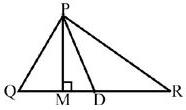Solution:

Median is a line joining the vertex and middle of the opposite side, and hence dividing the triangle in two equal parts. So PD is the median.

QUESTION: 7

The value of x in the adjoining figure is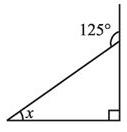Solution:
QUESTION: 8

What is the value of x in the below figure.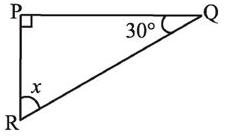Solution:
QUESTION: 9

Find the value of unknown x in the adjoining figure.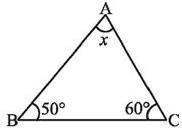Solution:
QUESTION: 10

What is the measure of angle x ?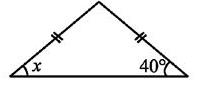Solution:
QUESTION: 11

ΔABC is right-angled at C. If AC = 5 cm and BC = 12 cm find the length of AB.

Solution:

AB2=AC2+BC2=52+122=25+144 = 169
AB=13 cm

QUESTION: 12

ΔPQR is a triangle right-angled at P. If PQ = 3 cm and PR = 4 cm, find QR.

Solution:

Using pythagoras theorem,
QR= PR+ PQ2
42+32
=16+9=25
QR = 5cm

QUESTION: 13

Which is the longest side in the triangle PQR right angled at P?

Solution:
QUESTION: 14

Which is the longest side in the triangle ABC right angled at B?

Solution:
QUESTION: 15

Which is the longest side of a right triangle?

Solution:
QUESTION: 16

What is the measure of angles x?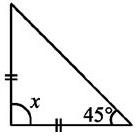Solution:

Since the two sides are equal, the angles opposite to them are also equal. So x+45° + 45° = 180°, x = 90°

QUESTION: 17

The value of x in the adjoining figure is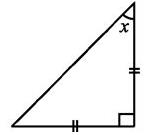Solution:
QUESTION: 18

A triangle in which all three sides are of equal lengths is called _________.

Solution:
QUESTION: 19

Find angle x in below figure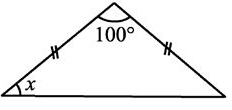Solution:
QUESTION: 20

Find angle x in below figure.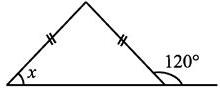Solution:

Since the two sides are equal, the angles opposite to them are also equal to the adjacent angle of 120° which is equal to 180° - 120° =60° is equal to x. So x = 60°